Test: Radiative Heat Transfer Level - 3

# Test: Radiative Heat Transfer Level - 3

Test Description

## 20 Questions MCQ Test Heat Transfer | Test: Radiative Heat Transfer Level - 3

Test: Radiative Heat Transfer Level - 3 for Chemical Engineering 2022 is part of Heat Transfer preparation. The Test: Radiative Heat Transfer Level - 3 questions and answers have been prepared according to the Chemical Engineering exam syllabus.The Test: Radiative Heat Transfer Level - 3 MCQs are made for Chemical Engineering 2022 Exam. Find important definitions, questions, notes, meanings, examples, exercises, MCQs and online tests for Test: Radiative Heat Transfer Level - 3 below.
Solutions of Test: Radiative Heat Transfer Level - 3 questions in English are available as part of our Heat Transfer for Chemical Engineering & Test: Radiative Heat Transfer Level - 3 solutions in Hindi for Heat Transfer course. Download more important topics, notes, lectures and mock test series for Chemical Engineering Exam by signing up for free. Attempt Test: Radiative Heat Transfer Level - 3 | 20 questions in 60 minutes | Mock test for Chemical Engineering preparation | Free important questions MCQ to study Heat Transfer for Chemical Engineering Exam | Download free PDF with solutions
 1 Crore+ students have signed up on EduRev. Have you?
Test: Radiative Heat Transfer Level - 3 - Question 1

### The dark surface of a ceramic stove top may be approximated as a blackbody. The “burners,” which are integral with the stove top, heated from below by electric resistance heaters.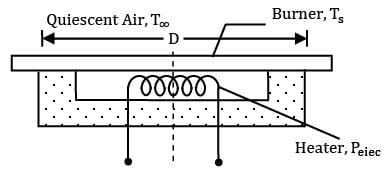Consider a burner of diameter D = 200mm operating at a uniform surface temperature of Ts = 250oC in ambient air at . Without a pot or pan on the burner, if the efficiency associated with energy transfer from the heaters to the burners is , what is the electric power requirement? (The relation between Nusselt number and Rayleigh number is given as NuL = (RaL)¼) Air (Tf = 408 K) k = 0.0344 x W/mK ν = 27.4 x 10-6 m2/s α = 39.7 x 10-6 m2/s Pr = 0.70 β = 0.00245 K-6

Detailed Solution for Test: Radiative Heat Transfer Level - 3 - Question 1

For emission from a black body,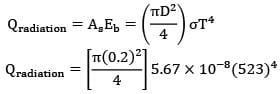= 13 W

∵ L = As/P = D/4 = 0.2/4 = 0.05 m

⟶ RsL = gβ(Ts-T)L3

= 9.81 x 0.00245 x 230 x (0.05)3/27.4 x 39.7 x 10-12

= 6.35 x 105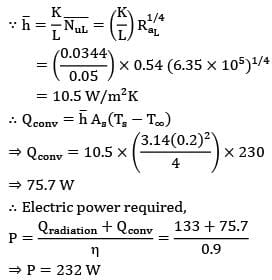= 10.5 W/m2 K

∴ Qconv = h As(Ts-T)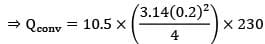⇒ 75.7 W

∴ Electric power required,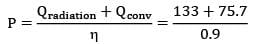⇒ P = 232 W

Test: Radiative Heat Transfer Level - 3 - Question 2

### A metal storage tank with planar surfaces of external area contains water at and is located inside a large enclosure with walls at 65oC . The tank is initially painted black on the outside and its emissivity is approximated to 0.95. How much percentage reduction in heat loss would occur if the outside surface of tank is coated with aluminium paint (instead of black) of emissivity 0.55? Take radiation constant σb = 5.67 x 10-8 W/m2K4

Detailed Solution for Test: Radiative Heat Transfer Level - 3 - Question 2

Consider outer surface of metal tank to be 1 & inner surface of enclosure to be surface 2.

By concept of thermal circuits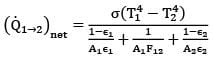As the surfaces of 1 are planar, F11 = 0

∴ F21 = 1

Also ,

A2 >> A1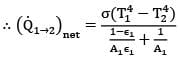∴ (Q1➝2)net = σA1ε1(T14 - T24)

∴ Q ∝ ε1

Q0.55/Q0.95 = 0.55/0.95 = 0.4211

Thus heat exchange rate decreases by 57.89 %, by painting the tank with aluminium paint.

Test: Radiative Heat Transfer Level - 3 - Question 3

### Two infinitely long cylinders are placed coaxially. The inner cylinder has an outside diameter of 7.5 cm, and emissivity of 0.1 and is maintained at 1050 K. The outer cylinder has an inside diameter of 22.5 cm, an emissivity of 0.2 and is maintained at 315 K. A cylindrical shield (very thin so that its temperature is the same on both sides) is placed concentrically midway between the two cylinders and allowed to come to thermal equilibrium. It has an emissivity of 0.3 on both sides. Assuming that vacuum exists between the annular spaces. Find the steady state temperature attained by the cylindrical shield.

Detailed Solution for Test: Radiative Heat Transfer Level - 3 - Question 3

Since the shield is placed midway between both the cylinders, therefore diameter of the shield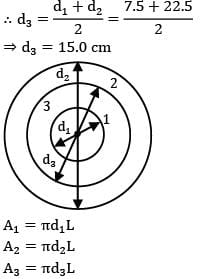∴ d3 = d1+d2/2 = 7.5 + 22.5/2

⇒ d3 = 15.0 cm

A1 = πd1L

A2 = πd2L

A3 = πd3L

For the system to be steady,

(Q1➝3)net = (Q3➝2)net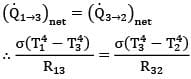By thermal circuits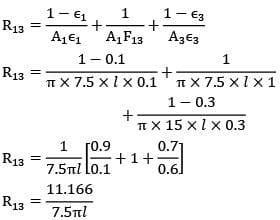R13 = 11.166/7.5πl

Similarly,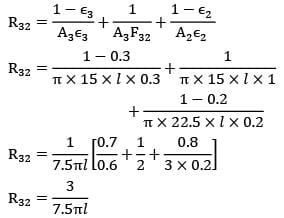R32 = 3/7.5πl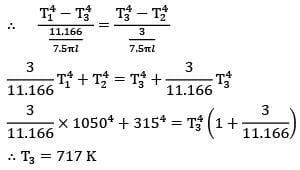∴ T3 = 717 K

Question_type 5

Test: Radiative Heat Transfer Level - 3 - Question 4

The spectral emissivity of an opaque surface at 800 K is approximated as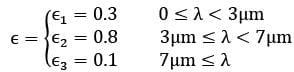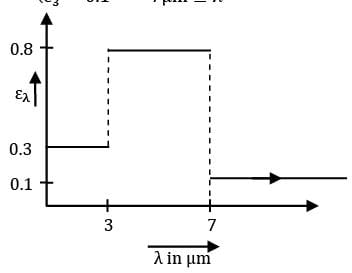Black body radiation function values for a black surface at 800 K are as follows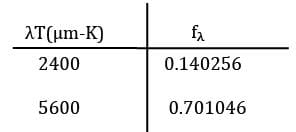Find the total emissivity of the surface?

Detailed Solution for Test: Radiative Heat Transfer Level - 3 - Question 4

By definition of total emissivity

ε = E/Eb

Where E =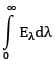∴ E =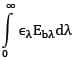∴ E =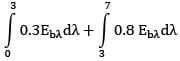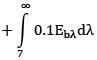∴ E = 0.3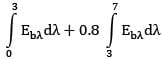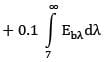E = 0.3 Eb,0➝3 + 0.8Eb,3➝7 + 0.1Eb,7➝ꝏ

E = 0.3 f3Eb + 0.8f3➝7Eb + 0.1f7➝ꝏE

E = [0.3f3 + 0.8(f7 -f3) + 0.1(1- f7)]Eb

∴ ε = [0.3f3 + 0.8(f7 - f3 ) + 0.1(1-f7)]

For T = 2400 μm-K

∴ f3 = 0.140256

Similarly for λ = 7 μm

λT = 5600 μm-K

∴ f7 = 0.701046

∴ ε = 0.3 x 0.140256 + 0.8

X (0.701046 - 0.140256) + 0.1 x (1 - 0.701046)

ε = 0.0420768 + 0.448632 + 0.0298954

ε = 0.5206042

≃ 0.52

Test: Radiative Heat Transfer Level - 3 - Question 5

Consider an infinitely long three sided triangular enclosure with sides 2 cm, 3 cm and 4 cm. The view factor from 2 cm side to 4 cm side is __________.

Detailed Solution for Test: Radiative Heat Transfer Level - 3 - Question 5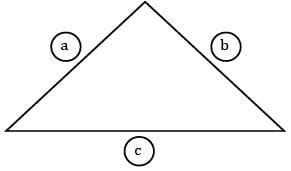Given la = 2 cm

Lb = 3 cm

Lc = 4 cm

By energyF conservation principle

Faa + Fab + Fac = 1

Fba + Fbb + Fbc = 1

Fca + Fcb + Fcc = 1

As a,b,c are planar surfaces

Faa, Fbb, Fcc, = 0

Therefore the equations are reduced to

Fab + Fac = 1 ….①

Fba + Fbc + = 1 ...②

Fca + Fcb = 1 ….③

By Reciprocity theorem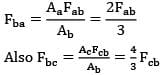Substituting Fba & Fbc in equation ②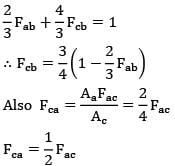Substituting Fcb & Fca in equation ③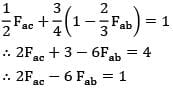∴ 2Fac - 6Fab = 1

Fac - 3Fab = 1/2 ….Ⓢ

Solving equation ① & Ⓢ together

Fab + Fac =1

Fac - 3Fab = 1/2

____________

3Fab + 3ac = 3

3Fac - 3Fab = 1/2

+ + +

______________

6Fac = 7/2

Fac = 7/12

Fac 0.5833

Question_type 5

Test: Radiative Heat Transfer Level - 3 - Question 6

An industrial furnace employs a hollow brick lining. The inside and outside surfaces of the hollow brick lining are maintained at 900 K and 430 K by placing radiation shields in between the hollow space. The heat loss occurs to the surroundings (300 K) by both radiation and natural convection. Calculate number of shields needed. The emissivity of walls and shields can be taken as 0.85. The convective heat transfer coefficient is governed by the expression

h = 1.5(ΔT)0.33 W/m2- K

Detailed Solution for Test: Radiative Heat Transfer Level - 3 - Question 6 Heat lost by outer surface of the lining to surroundings per unit area,

q = εσ(T24 - T4)

q = 0.85 x 5.67 10-8[4304 - 3004]+1.5(430 -300)0.33 x (430 - 300)

q = 1257.3116 + 971.932

q = 2229.24 W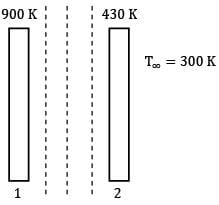n-shields

∴ Heat transfer from surface 1 to 2 through n-shields must be equal to ̇q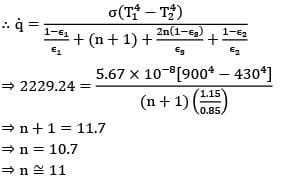⇒ 2229.24 =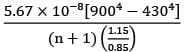⇒ n+1 = 11.7

⇒ n = 10.7

⇒ n ≅ 11

Question_type 5

Test: Radiative Heat Transfer Level - 3 - Question 7

An electrically heated industrial furnace cavity is modeled in the form of a cylinder having diameter 10 cm and length 20 cm. It is opened at one end to the surrounding, that is at a temperature of 300 K. The electrically heated sides and the bottom of the cavity which are well insulated and may be approximated as black bodies, maintained at a temperature of 1800 K and 2000 K respectively. Find the power required to maintain the cylindrical surface at this condition. Take shape factor from the bottom surface to surroundings as 0.06.

Detailed Solution for Test: Radiative Heat Transfer Level - 3 - Question 7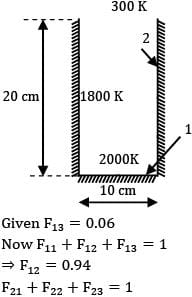Given F13 = 0.06

Now F11 +F12 + F13 = 1

⇒ F12 = 0.94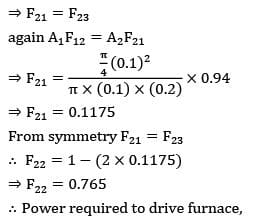⇒ F21 = F23

again A1F12 = A2F21

⇒ F21 = 0.1175

From Symmetry F21 = F23

∴ F22 = 1-(2 x 0.1175)

⇒ F22 = 0.765

∴ Power required to drive furnace,

= A2A21 σ(T24 - T14) + A2F23σ(T24 - T34)

= A2F21σ[2T24 - T14 - T34]

= 3.14(0.1)(0.2).0.1175 x 5.67

X 10-8[2 x (1800)4 - (2000)4 - 3004]

= 2.087 kW

Question_type 5

Test: Radiative Heat Transfer Level - 3 - Question 8

A furnace is shaped like a long equilateral triangular duct. The width of each side is 1 m. The base surface has an emissivity of 0.7 and is maintained at a uniform temperature of 600 K . The heated left-side surface closely approximates a blackbody at . The right-side surface is well insulated. Determine the rate in kW at which heat must be supplied to the heated side externally per unit length of the duct in order to maintain these operating conditions.

Detailed Solution for Test: Radiative Heat Transfer Level - 3 - Question 8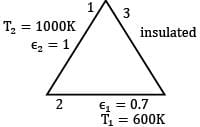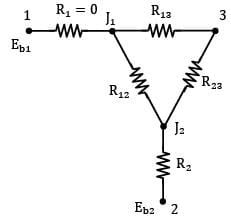Since the surface 3 is a reradiating surface, (R3 = 0) the thermal circuit can be drawn as shown above. Since surface 1 is a black surface,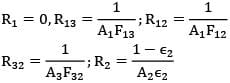∵ Length of all sides is equal to 1 m and taking unit length of the duct

A1 = A2 = A3 = 1 x 1 = 1 m2

Total thermal resistance between 2 and 2 is,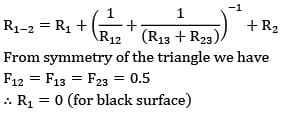From symmetry of the triangle we have

F12 = F13 = F23 = 0.5

∴ R1 = 0 (for black surface)

R12 = 1/A1F12 = 1/1 x 0.5 = 2

R13 = 1/A1F13 = 1/1 x 0.5 = 2

R23 = 1/A2F23 = 1/1 x 0.5 = 2

R2 = 1-ε2/A2ε2 = 1-0.7/1 x 0.7 = 0.43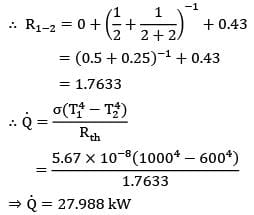= (0.5 + 0.25)-1 + 0.43

= 1.7633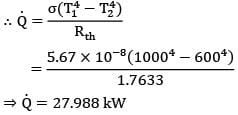= 5.67 x 10-8(10004 - 6004)/1.7633

Q = 27.988 kW

Question_type 5

Test: Radiative Heat Transfer Level - 3 - Question 9

Air is flowing inside a cylindrical tube in which a thermocouple is kept. Convective heat transfer coefficient between air and thermocouple is 60 W/m2- C, εthermocouple = 0.5, εwall = 0.6. If Ta = 970o C and Tc = 800oC then, Tw is ________. Air is a non-participating medium in terms of radiation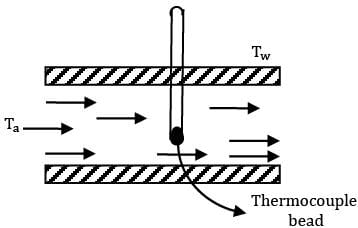(Tc)

Option~

(A) 1041.7oC

(B) 1000oC

(C) 768.7oC

(D) 509oC

Detailed Solution for Test: Radiative Heat Transfer Level - 3 - Question 9 For the given arrangement to be in steady state, heat convection from air to thermocouple

= heat radiation from thermocouple to walls

∴ hAT/C(TA - TC) = εT/C(TC4 - Tw4). σ

(∵ Area of walls very large as compared to area of thermocouple)

60 x 70 = 0.5 x 5.67 x 10-8(10734 - Tw4)

10734 - Tw4 = 1.4815 x 1011

Tw = 1041.67 K

Tw = 768.7oC

Test: Radiative Heat Transfer Level - 3 - Question 10

If is the emissivity of surfaces and shields and n is the number of shields introduced between the two surfaces, then overall emissivity is given by

Option~

(A) n/ε

(B) 1/(2n-ε)

(C) nε

(D) ε/[(n+1)(2-ε)]

Detailed Solution for Test: Radiative Heat Transfer Level - 3 - Question 10 Total resistance between two surfaces having n shields in between them is given by,

Rth = (n+1)(2/ε -1) (for unit area)

Overall resistance of a surface is given by,

Rth = 1/εo (for unit area)

Where εo ￫ overall emissivity

∴ 1/εo = (n+1)(2/ε -1)

1/εo = 2n/ε - n + 2/ε - 1

1/εo = 2n - nε + 2-ε/ε

1/εo = n(2-ε) + 1( 2-ε )/ε

1/εo = (n+1)(2-ε)/ε

εo = ε/(n+1)(2-ε)

Test: Radiative Heat Transfer Level - 3 - Question 11

The net radiation from the surfaces of two parallel plates maintained at is to be reduced by at least 99%. Number of shields which should be placed between surfaces to achieve this reduction is (εshield = 0.05), (εsurface = 0.8)

Option~

(A) 3

(B) 4

(C) 5

(D) 6

Detailed Solution for Test: Radiative Heat Transfer Level - 3 - Question 11 By thermal circuit

Q ∝ 1/R

where R is the resistance to radiation heat exchange between the plates. When there is no shield

R1 = 1-ε1/Aε1 + 1/A + 1-ε2/Aε2

R1 = 1-0.8/A x 0.8 + 1/A + 1-0.8//A x 0.8

R1 = 1.5/A

When n shields are placed in between the plates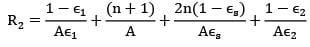= 1.5 + 39 n/A

Based on given condition

Q2/Q1 = 0.01

∴ 1.5/1.5 + 39 n = 0.01

∴ n = 3.8

i.e.n ≃ 4

Test: Radiative Heat Transfer Level - 3 - Question 12

In the previous question if εshield = εsurface, then what will be the number of shields?

Option~

(A) 100

(B) 3

(C) 99

(D) 4

Detailed Solution for Test: Radiative Heat Transfer Level - 3 - Question 12 With introduction of n shields the rate of heat transfer reduces by 99%, therefore,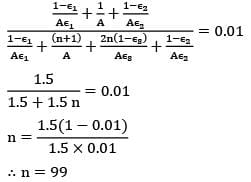1.5/1.5+1.5n = 0.01

n = 1.5(1-0.01)/1.5 x 0.01

n = 99

This shows that if the emissivity of the surface is large then number of shields should be large as shield shall offer less resistance. Hence for shields to be effective their surface emissivity should be as low as possible.

Test: Radiative Heat Transfer Level - 3 - Question 13

Consider sun (radius R) to be a black body of temperature T. Find out the view factor of earth ( with respect to sun. The center to center distance between sun and earth is (l >>r,R).

Option~

(A)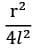(B)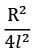(C)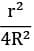(D)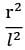Detailed Solution for Test: Radiative Heat Transfer Level - 3 - Question 13 By definition of view factor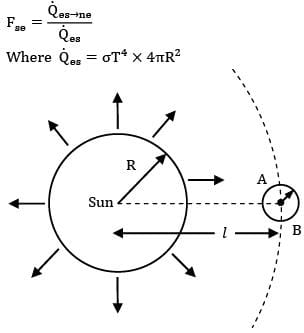Fse = Qes➝ne/Qes

Where Qes = σT4 x 4πR2

Consider an imaginary sphere of radius l with its center at center of sun. As sun is a symmetric body it emits radiation uniformly in all direction which is received uniformly at surface of imaginary sphere. The energy received by earth is actually the energy to be received by AB portion of the surface of imaginary sphere, where AB is interaction of imaginary sphere and earth. l >> r As therefore AB is nearly a circular surface of area πR2.

Total energy emitted by sum = σ(4πR2)T4

∴ Energy received per unit area of imaginary sphere

= σ(4πR2)T4/4πl2

= σR2T4/l2

∴ Energy received by earth (i.e. surface AB)

= σR2T4/l2 x πr2

Hence Fse =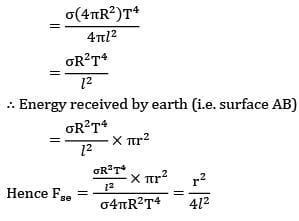*Answer can only contain numeric values
Test: Radiative Heat Transfer Level - 3 - Question 14

A cake is being baked in an hemispherical oven of radius 500 mm. The lower surface of the oven is maintained at 1000 K while the covering surface ( is maintained at 800 K. The diameter of the round cake is 0.25 m and its height can be neglected. Considering the lower surface of the oven and cake to be black bodies and neglecting the area covered by the cake, find the time required to bake the cake, if it requires 3.2123 MJ.

Detailed Solution for Test: Radiative Heat Transfer Level - 3 - Question 14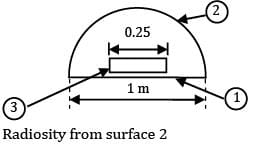= reflected energy from ② + emitted energy from ②

⇒ J2 = ρ2σA1T14 + ε2 A2 σT24

Ε2 = 0.8 ⇒ α2 = 0.8

∴ α222 = 1

⇒ ρ2 = 0.2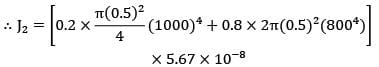⇒ J2 = 38,071.644 W

Energy incident on the cake, G3 = F23J2

A3F32 = A2F23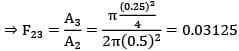∴ G3 = 0.03125 x 38071.644

G3 = 1189.74 W

∴ Time required to bake the cake,

t = 3.2123 x 106/1189.74

⇒ t = 2700 s

⇒ t ≅ 45 min

Test: Radiative Heat Transfer Level - 3 - Question 15

Determine the shape factor between a small area and a parallel circular disc A2(diameter D) is located on the axis of the disc and the semi-vertex angle of the cone formed with the disc as base and as the vertex is α.

Option~

(A)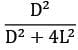(B)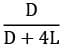(C)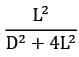(D)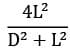Detailed Solution for Test: Radiative Heat Transfer Level - 3 - Question 15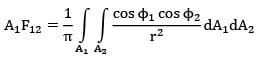Considering an elementary ring dA2 of width dr at a radius r,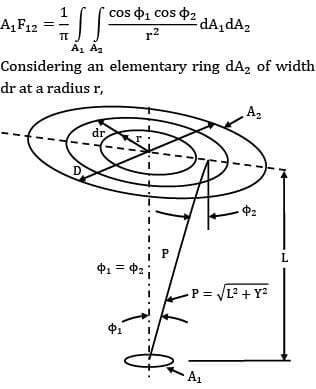dA2 = 2 πr dr

Now, r = L tanΦ1

Dr = L sec2Ф11

dA2 = 2πL2 tan Φ1 sec2 Φ11

L/P = cos Φ1 or P = L/cosΦ1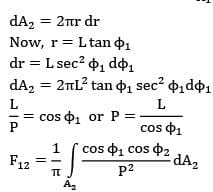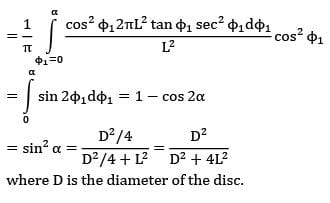= sin2 α = D2/4/D2/4 + L2 = D2/D2 + 4L2

where D is the diameter of the disc.

Test: Radiative Heat Transfer Level - 3 - Question 16

A small surface of area emits radiation as a blackbody at temperature T1 = 600 K. A fraction of energy emitted by A1 strikes another small surface of area A2 = 5 cm2 oriented as shown in the figure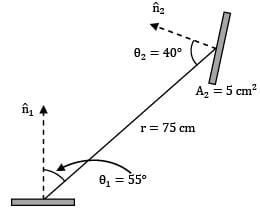A1 = 3 cm2

T1 = 600 K

Find out view factor of surface with respect to A1 ?

Option~

(A) 0.6215 x 10-4

(B) 1.234 x 10-4

(C) 4.14 x 10-4

(D) 2.071 x 10-4

Detailed Solution for Test: Radiative Heat Transfer Level - 3 - Question 16 View factor (F12 ) is the fraction of energy leaving the surface that is received at surface But as is a black surface, energy leaving the surface is only the emitted radiation.

∴ F12 = qc1➝r2/Qc1

By Stefan-Blotzmann law

Qc1 = σA1T14

Also, using the definition of Intensity of emitted radiation,

Qc1➝r2 = Ic1A1 cos ፀ1ω2➝1

Where ω2➝1 is solid angle subtended by point surface 2 over point surface 1.

∴ ω2➝1 = A2cosፀ2/r2

For black surface

Ic1 = σT14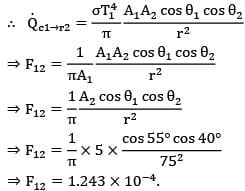⇒ F12 = 1.243 x 10-4

*Answer can only contain numeric values
Test: Radiative Heat Transfer Level - 3 - Question 17

A small surface of area emits radiation as a blackbody. A fraction of this energy emitted is received by another small area Oriented as shown in figure.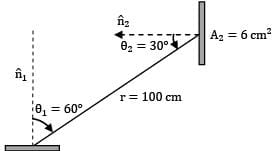A1 = 2 cm2

The rate at which radiation emitted by surface 1 strikes is measured to be 274 x 10-6 W. Determine the surface temperature of in K?

Detailed Solution for Test: Radiative Heat Transfer Level - 3 - Question 17 As surface 1 is a black surface, the energy leaving the surface is only the energy emitted by the surface.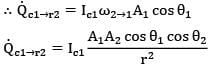Also by definition of black surface (diffuse characteristics)

Ic1 = σT14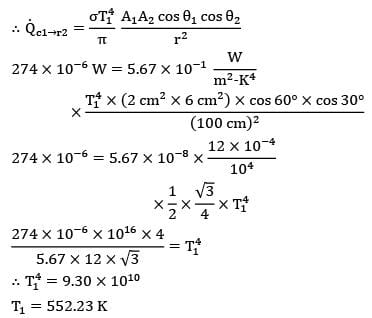274 x 10-6 W = 5.67 x 10-1 W/m2- K4 x T14 x ( 2cm2 x 6 cm2) x cos 60o x cos 30o /(100 cm)2

274 x 10-6 = 5.67 x 10-8 x 12 x 10-4/ 104 x 1/2 x ✓3/4 x T14

274 x 10-6 x 10-8 x 4 / 5.67 x 12 x ✓3 = T14

∴ T14 = 9.30 x 1010

T1 = 552.23 K

Test: Radiative Heat Transfer Level - 3 - Question 18

A small stationary surface of area A1 = 10-4m2 emits diffusely with a total intensity of . A second surface of equivalent area is located at a fixed distance from . The connecting line between the two surfaces remains perpendicular to while rotates at an angular frequency of

2 = dፀ2/dt = 2 rad/s about the axis as shown in the figure.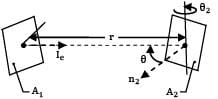What is the amount of energy intercepted by the two sides of , during one complete revolution?

Option~

(A) 2 x 10-6j

(B) 4 x 10-6j

(C) 8.0 x 10-6j

(D) 16 x 10-6j

Detailed Solution for Test: Radiative Heat Transfer Level - 3 - Question 18 At time instant t the normal to surface of plate 2 which faces plate 1 makes an angle of ፀ2 with the direction of view ®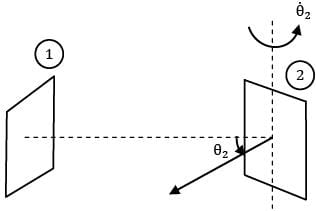Thus for the given time instant the rate at which energy emitted by 1 strikes at 2 is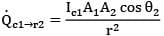Thus for the given time instant the rate at which energy emitted by 1 strikes at 2 is

dQ = Qc1➝r2 dt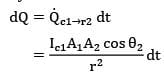Where,

dt = dፀ22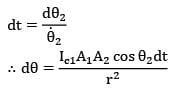This face of the surface 2 is in front of surface

1 for θ2 = π/2 to π/2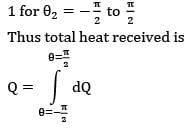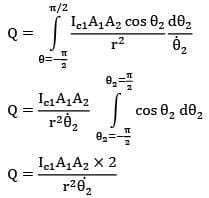Similarly second face of surface 2 will also come in front of surface 1 during remaining half of revolution Thus total energy received by surface 2 during one complete revolution is

4Ic1A1A2 / r22

= 4 x 100 x 10-4 x 10-4/(0.5)2 x 2

= 8 x 10-6 j

*Answer can only contain numeric values
Test: Radiative Heat Transfer Level - 3 - Question 19

On an overcast day the directional distribution of the solar radiation incident on the earth’s surface may be approximated by an expression of the form where In = 80 W/m2is the total intensity of radiation directed normal to the surface and is the zenith angle. What is the solar irradiation at the earth’s surface?

Detailed Solution for Test: Radiative Heat Transfer Level - 3 - Question 19 Consider a point on the earth’s surface receiving solar radiation from all hemispherical directions above the point.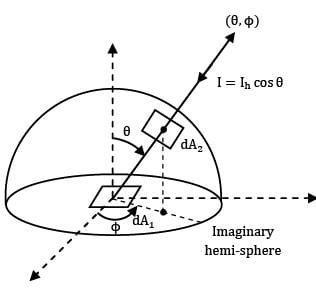By definition of intensity of radiation

dQ1 = I(dA1 cos ፀ)ωdA2➝dA1 where dA2 is a small area on hemispherical surface.

∴ ωdA2➝dA1 = (rsin ፀ dф)(rdፀ)/r2

= sin ፀ dፀ dф

∴ dQ1 - IdA1 sin θ dθ dф

dQ1 - Incos ө dA1 sin θ dθ dф

dQ1 - IndA1 sin 2θ/2 x dθ dф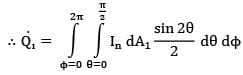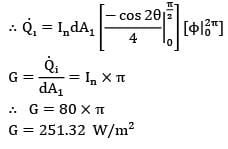G = Qi/dA1 =In x π

∴ G = 80 x π

G = 251.32 W/m2

Test: Radiative Heat Transfer Level - 3 - Question 20

The spectral, hemispherical absorptivity of an opaque surface and the spectral irradiation at the surface are as shown.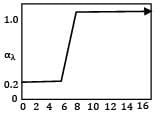λ(μm)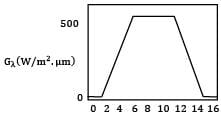λ(μm)

What is the total hemispherical absorptivity of the surface?

Detailed Solution for Test: Radiative Heat Transfer Level - 3 - Question 20 Based on data given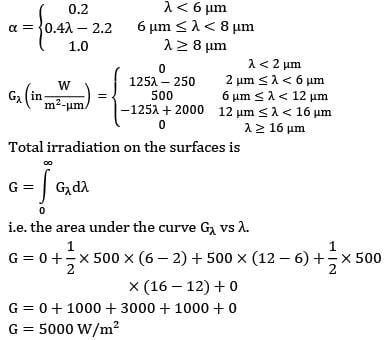Total irradiation on the surface is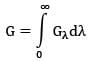i.e. the area under the curve Gλ vs λ.

G = 0+1/2 x 500 x (6-2) + 500 x (12-6) + 1/2 x 500 x (16-12) + 0

G = 0 + 1000 + 3000 + 1000 + 0

G = 5000 W/m2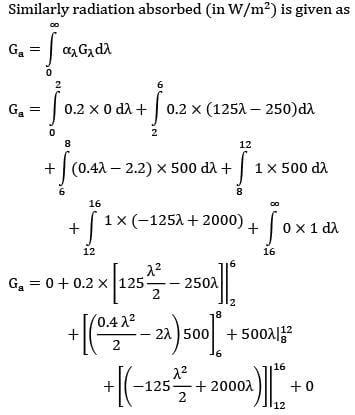Similarly radiation absorbed (in W/m2 ) is given as

Ga = 1000+800+2000+100

Ga = 4800 W/m2

∴ α = Ga/G

α = 4800/5000

α = 0.96

Quesiton_type: 5

## Heat Transfer

58 videos|70 docs|85 tests
 Use Code STAYHOME200 and get INR 200 additional OFF Use Coupon Code